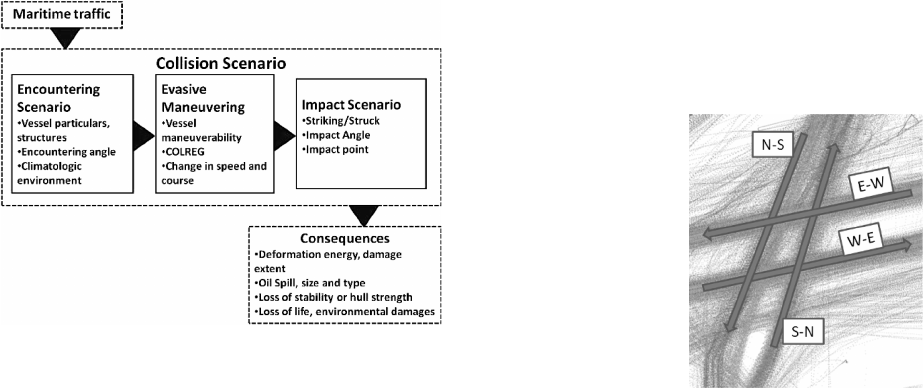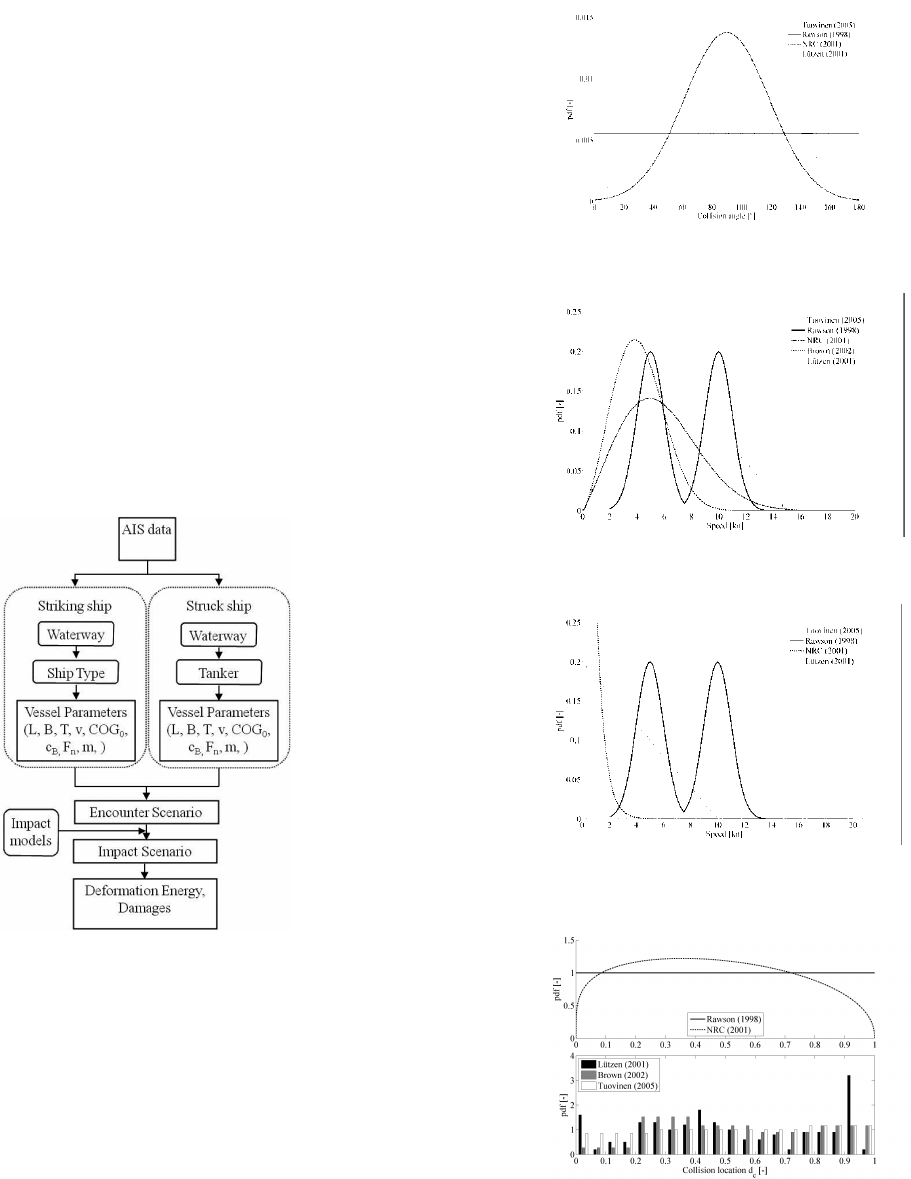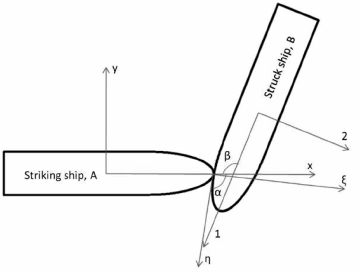International Journal
and Safety of Sea Transportation
Volume 6
Number 1
March 2012
47
1 INTRODUCTION
Ship-ship collisions are rare events that potentially
might have disastrous impact on the environment,
human life and economics. To find effective risk
mitigating measures the risk must be reliably as-
sessed. Proper assessment of the ship-ship collision
risk requires understanding on the complicated chain
of events. Simplifying assumptions on certain pa-
rameters are necessary as the research in this field is
not comprehensive. Especially, the important link
between the encounter of the colliding vessels and
the actual moment of impact contain obvious uncer-
tainties.
In this paper a case study is conducted to compare
models found in literature for dynamic parameters in
collision scenario. The case study concerns colli-
sions in which the struck vessel is an oil tanker. The
traffic is simulated by means of a Monte Carlo simu-
lation based on AIS data to obtain realistic encounter
scenarios for the analyzed area. The assumptions are
then applied to encounter scenario to obtain the
complete impact scenario. The deformation energy
released in the collision is calculated by analytic
method (Zhang 1999) and the damage extents are es-
timated with simple formula to normalize the results
of deformation energy calculations. The effects of
assumptions for dynamic parameters to collision risk
are discussed.
2 COLLISION RISK EVALUATION
2.1 Concept of risk
Risk is a product of probability p and consequences
c and is expressed with (Kujala et al, 2010)
=
i
c
i
pR
(1)
where i denotes certain chain of events or scenario.
2.2 Tanker Collisions
In case of ship-ship collisions scenario is a function
of vast number of static and dynamic parameters.
The parameters used in this study are listed in Ta-
ble 1.
Uncertainty in Analytical Collision Dynamics
Model Due to Assumptions in Dynamic
Parameters
K. Ståhlberg, F. Goerlandt, J. Montewka* & P. Kujala
Aalto University, School of Engineering, Department of Applied Mechanics, Marine
Technology, Espoo, Finland
* Aalto University, School of Engineering, Marine Technology, Espoo, Finland
Maritime University of Szczecin, Institute of Marine Traffic Engineering, Poland
ABSTRACT: The collision dynamics model is a vital part in maritime risk analysis. Different models have
been introduced since Minorsky first presented collision dynamics model. Lately, increased computing capac-
ity has led to development of more sophisticated models. Although the dynamics of ship collisions have been
studied and understanding on the affecting factors is increased, there are many assumptions required to com-
plete the analysis. The uncertainty in the dynamic parameters due to assumptions is not often considered.
In this paper a case study is conducted to show how input models for dynamic parameters affect the results of
collision energy calculations and thus probability of an oil spill. The released deformation energy in collision
is estimated by the means of the analytical collision dynamics model Zhang presented in his PhD thesis. The
case study concerns the sea area between Helsinki and Tallinn where a crossing of two densely trafficked wa-
terways is located. Actual traffic data is utilized to obtain realistic encounter scenarios by means of Monte
Carlo simulation. Applicability of the compared assumptions is discussed based on the findings of the case
study.48
Table 1. Collision parameters used in this study.
________________________________________________
Description Unit Type
________________________________________________
M Mass [kg] Static
L Length [m] Static
B Width [m] Static
m
x
surge motion
m
y
sway motion
j Added mass coefficient, [-] Static
rotation around centre of gravity
R Radius of ship mass inertia [m] Static
around centre of gravity
V
x
Surge speed [m/s] Dynamic
V
y
Sway speed [m/s] Dynamic
x x-position of centre of gravity [m] Static
y y-position of centre of gravity [m] Static
x
c
x-position of impact point, [m] Dynamic
in coordinate system ship A
y
c
y-position of impact point, [m] Dynamic
in coordinate system ship A
μ
0
coefficient of friction [-] Static
e coefficient of restitution [-] Static
________________________________________________
The static parameters can be derived from AIS
data, statistics and theory of ship design. Modeling
of the dynamic parameters is often based on statis-
tics of the collisions.
Ship-ship collision risk evaluation schematic is
outlined in Figure 1 for the case of an oil tanker be-
ing struck vessel.
Figure 1. Tanker collision risk evaluation schematic
The first step of the risk analysis is modeling the
traffic in the analyzed area. Modeling may be done
via simulation of individual vessel movements as
proposed by Merrick et al. (2003), van Dorp et al.
(2009), Ulusçu et al. (2009) and Goerlandt & Kujala
(2010) or alternatively by simulating the traffic
flows as proposed by Pedersen (1995, 2010) or
Montewka et al (2010). The encounter scenarios are
obtained as a result of the traffic simulation. The
impact scenarios may be then obtained with the
models discussed in detail in Section 3.3.
Second part of the risk analysis is the evaluation
of the consequences which begins with the estimat-
ing the released deformation energy that is absorbed
by the vessel structures. Collision dynamics models
to calculate the deformation energy can be divided
into two groups, time domain and analytical (Wang
et al 2000), based on applied calculation method.
Analytical closed form methods have been proposed
by Minorsky (1959), Vaughan (1977), Hutchison
(1986), Hanhirova (1995), and Zhang (1999). Mod-
els based on time domain calculations are proposed
by Chen (2000) and Tabri et al. (2009). In analytical
models the external dynamics and internal mechan-
ics are uncoupled while in time domain methods
these are coupled.
3 COMPARISON METHODS
3.1 Traffic simulation and encounter scenarios
The traffic simulation is described here shortly as
the simulation itself is not crucial regarding the
comparison of impact models. The simulation is de-
scribed in detail in (Ståhlberg, 2010)
The traffic in the analyzed area is obtained from
AIS data. The data contains traffic information from
the month of July 2006 in the sea area between Hel-
sinki and Tallinn where densely trafficked water-
ways cross. In Figure 2 the analyzed area and the da-
ta points are presented. The four main waterways in
the crossing area are named after compass quarters
in form of “from-to” as shown in Figure 2. The con-
sidered waterway combinations and resulting en-
counter types are listed with reference numbers in
Table 2.
Figure 2. Plot of AIS data points in analyzed area
The AIS data is filtered to distinguish the traffic
between waterways and ship types. The numbers of
passages through the analyzed area per ship type are
listed in Table 3. The Monte Carlo simulation
flowchart starting from the filtered AIS data is
shown in Figure 3. The result of the simulation is the
encounter situations based on the traffic data.49
Table 2. The considered waterway combinations and resulting
encounter types with respective reference numbers.
__________________________________________________
Ref number Route Encounter type
__________________________________________________
1 N-S, E-W Crossing
2 N-S, W-E Crossing
3 S-N, E-W Crossing
4 S-N, W-E Crossing
7 E-W, E-W Overtaking
8 W-E, W-E Overtaking
_________________________________________________
Table 3. Number of passages per ship type and route.
__________________________________________
Ship Route
_________________________________
Type N-S S-N E-W W-E
__________________________________________
HSC 741 740 0 0
PAX 253 254 26 14
Cargo 5 4 768 742
Tanker 0 0 218 215
Other 3 3 36 35
__________________________________________
* HSC = High Speed Craft, PAX = Passenger vessel,
Cargo = Cargo vessel
The Monte Carlo simulation to create encounter
scenarios is run 10000 times for those combinations
of main waterways in which the tanker may be
struck vessel. In the utilized data set tankers were
recorded sailing only on “E-W” and “W-E” water-
ways. In this study the probability of a vessel in-
volved in collision is weighted with the number of
voyages in the area.
Figure 3. Flowchart of Monte Carlo simulation
3.2 Impact scenario simulation
With the encountering vessels’ characteristics
known the impact scenarios are simulated here by
applying the compared models for the dynamic pa-
rameters. The models may be considered to be the
“evasive maneuvering” model shown in Figure 1.
The compared assumptions are presented in Fig-
ures 4-7 and the distribution parameters are com-
piled into Table 4.
In “Blind Navigator” model there are no maneu-
vering actions taken to avoid the collision and thus
the speeds and courses are unchanged from the en-
counter scenario. The collision location is assumed
to be uniformly distributed along the struck vessel’s
length. This model is used by Van Dorp & Merrick
(2009) and COWI(2008). Based on the analysis of
collisions in (Cahill, 2002) and (Buzek & Holdert,
1990) it seems extremely rare that neither vessel
takes any action.
Figure 4. Input distributions for collision angle, Lützen: initial
angle 90°, Brown (2002) quasi-equivalent to NRC (2001)
Figure 5. Input distributions for striking ship speed, Lützen
with initial speed of 15 kn
Figure 6. Input distributions for struck ship speed, Lützen with
initial speed of 10 kn, Brown (2002) quasi-equivalent to NRC
(2001)
Figure 7. Input distributions for location of impact along struck
ship’s length, 0 = aft, 1 = fore50
Table 4. Overview of impact scenario models.
__________________________________________________
Impact Collision V
A
V
B
Impact
Model Angle, β Point, d
[deg] [kn] [kn] [x/L]
__________________________________________________
Blind β=β
0
V
A
=V
A0
V
B
=V
B0
U(0,1)
Navi
Rawson U(0,180) bi-normal idem to U(0,1)
(1998) N(5,1) V
A
N(10,1)
Truncated {2, 14}
NRC N(90,29) W(6.5,2.2) E(0.584) B(1.25,1.45)
(2001) {0, 1}
Lützen T(0,β
0
,180) U(0,0.75V
A0
) T(0,V
B0
) Empirical
(2001) T(0.75V
A0
,V
A0
) See FIG 7
Brown N(90,29) W(4.7,2.5) E(0.584) Empirical
(2002) See FIG 7
Tuovinen Empirical Empirical Empirical Empirical
(2005) See FIG 4 See FIG 5 See FIG 6 See FIG 7
_________________________________________________
* Distributions are marked as follows, U=Uniform(min, max)
N=Normal(μ, σ), T=Triangular(min, triangle tip, max),
E=Exponential(λ), B=Beta(α, β, min, max), W=Weibull(k, λ)
Lützen’s (2001) set of assumptions implies that
the struck vessel is more prone to speed reduction
than the striking vessel while the impact angle is tri-
angularly distributed between 0° and 180° with the
tip of the distribution at the encounter angle. The
longitudinal impact location is given by empirical
distribution. Although there is no explanation how
the distributions for collision angle and velocities are
derived these are included into the comparison be-
cause of the existing relation between encounter and
impact scenarios.
Rawson et al (1998) model is based on statistics
of the grounding accidents with assumption that the
collision speed being similarly distributed as
grounding speed. Velocities of the colliding vessel
are distributed according to a double normal distri-
bution in which the averages are described to repre-
sent the service speed, i.e. no speed reduction, and
half of service speed. The same speed distribution is
used for both striking and struck vessel. Collision
angle and collision location are uniformly distribut-
ed between 0°…180° and along the struck vessel’s
length respectively.
Tuovinen (2006) compiled statistics from over
500 collisions. Statistics have been used here as pre-
sented originally, in form of empirical distributions.
Brown (2002) and NRC (2001) give quite similar
distributions. Brown gives lower velocity for the
striking vessel. These models both assume that strik-
ing vessel has higher velocity than struck at the mo-
ment of impact. It is noteworthy that these two mod-
els suggest much lower collision speeds than other
models. Collision angle is normally distributed
around right angle. In NRC model the collision loca-
tion is beta distributed so that midship section is
rammed at higher probability than the fore and aft of
the vessel while Brown suggests empirical distribu-
tion.
Overall, the distributions Lützen suggested are
the only ones taking the encounter into account in
any way and other models give same distributions
for dynamic parameters irrespective of encounter
scenario. None of these models indicate how to de-
termine which vessel is striking and which is struck.
It is assumed here that the probabilities of vessel be-
ing striking or struck are equal for all models as no
other probabilities were suggested in these models.
The compared models do not have the possibility of
initial sway nor yaw speeds, which in case of ma-
neuvering is unlikely.
It can be seen in the Figures 4-7 that models, with
exception of Brown and NRC, give distinctively dif-
ferent distributions for the dynamic parameters.
Considering the struck vessel speed being lower in
all the models expect Rawson it appears likely that
the collision statistics from which the distributions
are derived include collisions in which the struck
vessel is in anchorage or in berth. Tuovinen’s (2005)
statistics include approximately 6% of such cases
and 41% of open seas collisions. Brown (2002)
states that the share is significant as in about 60% of
collisions struck vessel speed is zero.
3.3 Deformation energy calculations
Zhang presented in his PhD thesis (Zhang, 1999) a
simplified calculation method for released
deformation energy in ship-ship collision. Zhang’s <